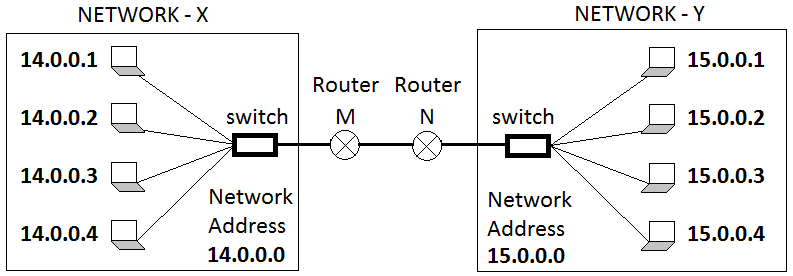Networking

The first address of any block is called the network address. It is used to identify the block in the Internet and so it is not assigned to any host on that particular block. Thus a network address has all the hostid bit set to 0.

For example, if we consider a class A IP address 14.28.42.56

By looking at the IP address we can say that the netid is 14 and the host id is 28.42.56 and the netid tells us that this IP address belongs to the 15th block of Class A (this is because the 1st block of Class A has netid 0).

So to find the network address all we have to do is copy the netid as is and set all the hostid to 0. So for the above IP address we will have the following network address: 14.0.0.0

Network address is used in routing a packet to the destination network. To understand this let us consider 2 networks as shown in the picture below.We have two networks X and Y and 4 hosts in each of the networks. The network address X and Y is 14.0.0.0 and 15.0.0.0 respectively.

Consider user A (14.0.0.1) of network X who sends a packet to user B (15.0.0.1) of network Y. The packet that A sends has the sender address (14.0.0.1) and the destination address (15.0.0.1). When the packet leaves A’s machine, it first goes to the router M which calculates the destination network address using masking (We will discuss masking in a moment) and forwards the packet to router N which sends the packet to B.

This is similar to postal system. Let us consider two metropolitan cities Kolkata and Mumbai and two friends Mr. Ganguly and Mr. Khan who lives in Kolkata and Mumbai respectively. If Mr. Khan writes a letter to his friend Mr. Ganguly it is first send from Mr. Khan’s address to Mumbai Post Office. On reaching the Mumbai PO the destination address is checked which is Kolkata, so it is forwarded to Kolkata Post Office. On reaching Kolkata PO the letter is send to Mr. Ganguly’s address.

Here the network address is Mumbai PO and Kolkata PO, while the user A and B are Mr. Khan and Mr. Ganguly respectively.

Question

If the IP address is given as 14.196.168.5, find the network address, last address and number maximum number of host in the network?

Given:

This is a Class A address.

So the netid is 14 and the hostid is 196.168.5

We get the network address by setting the hostid bits to 0.

We get the last address of the network by setting the hostid bits to 1.

Number of bits occupied by hostid = 24

Therefore, maximum number of host in this network = 224 = 16,777,216

To find the network address (the first address of any network) from any IP address we use network mask in classful addressing. Network mask is a 32 bit number in which the n leftmost bits are set to 1 while the remaining (32 – n) bits are set to 0. Value of n is equal to the number of bits used in the netid for the class to which the given IP address belongs.

 CLASS n Binary notation 32 bits Dotted Decimalnotation A 8 1111 1111 0000 0000 0000 0000 0000 0000 255.0.0.0 B 16 1111 1111 1111 1111 0000 0000 0000 0000 255.255.0.0 C 24 1111 1111 1111 1111 1111 1111 0000 0000 255.255.255.0

Network Address from a given IP address can be found by performing the AND operation.

 Truth Table A B A AND B 0 0 0 0 1 0 1 0 0 1 1 1

We can find the Network Address from binary notation and dotted decimal notation.

### Binary Notation

In this case the IP Address and the Network Mask is represented is binary form and then AND operation is performed to get the Network Address.

Example

### Dotted-Decimal Notation

In this case IP Address and the Network Mask is represented is dotted decimal form and then AND operation is performed to get the Network Address.

This is a shortcut method.

Step 1. Copy all the decimal number of IP address that are above 255 of Network Mask.

Step 2. Set the numbers to 0 that are above 0 of Network Mask.

Example

This is of Class A so Network Mask = 255.0.0.0

So we copy 14 directly as it is above 255 and set rest of the numbers to 0 as they are above 0 of Network Mask.

So the network address = 14.0.0.0

Example